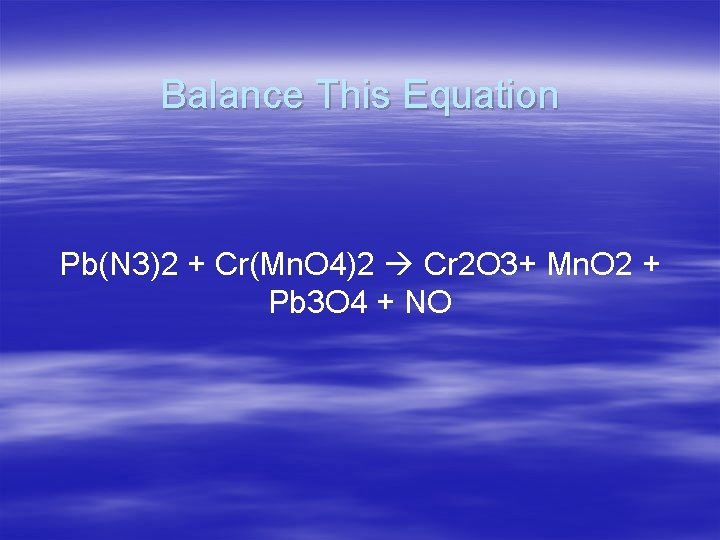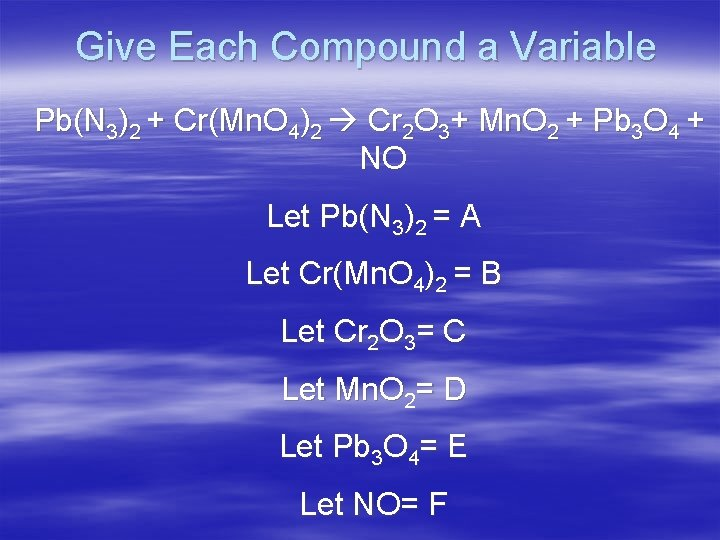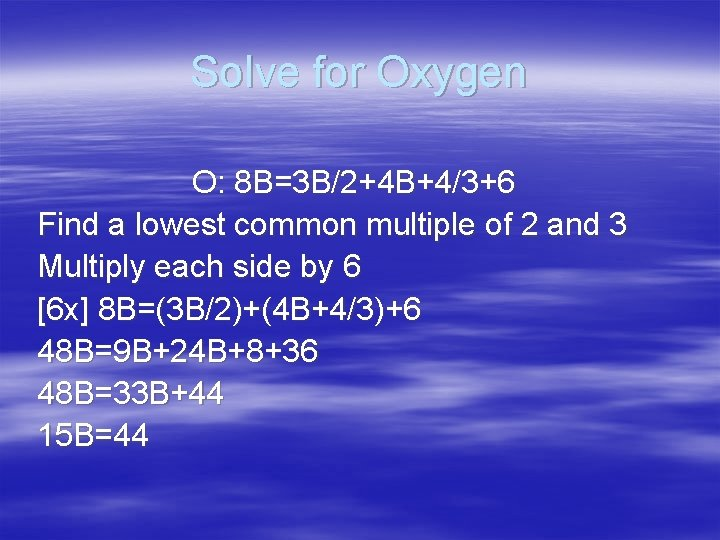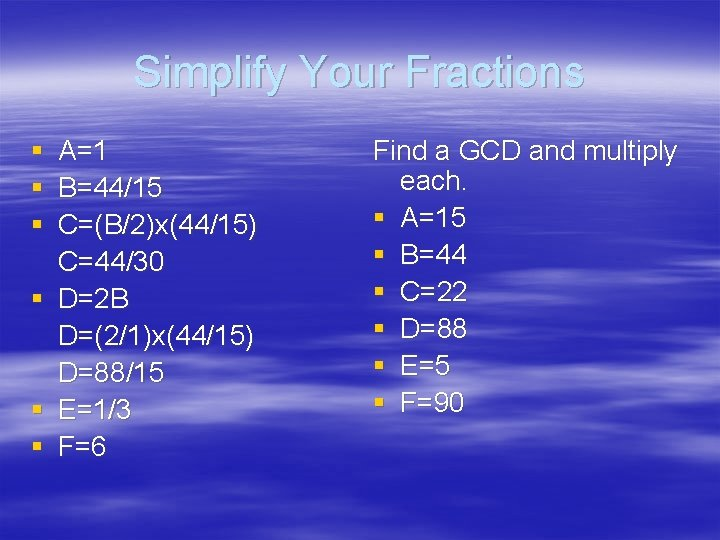Algebraic Solving Method Balancing Chemical Equations Balance This

• Slides: 9Algebraic Solving Method Balancing Chemical EquationsBalance This Equation Pb(N 3)2 + Cr(Mn. O 4)2 Cr 2 O 3+ Mn. O 2 + Pb 3 O 4 + NOGive Each Compound a Variable Pb(N 3)2 + Cr(Mn. O 4)2 Cr 2 O 3+ Mn. O 2 + Pb 3 O 4 + NO Let Pb(N 3)2 = A Let Cr(Mn. O 4)2 = B Let Cr 2 O 3= C Let Mn. O 2= D Let Pb 3 O 4= E Let NO= FSolve For Each of the Variables Pb(N 3)2+Cr(Mn. O 4)2 Cr 2 O 3+Mn. O 2+Pb 3 O 4+ NO Let A=1 § Pb: A=3 E * A is the amount of Pb on the left side of the equation *3 E is the amount on the left sideSolve For Each of the Variables Pb(N 3)2+Cr(Mn. O 4)2 Cr 2 O 3+Mn. O 2+Pb 3 O 4+NO § A=1 Pb: A=3 E N: 6 A=F Cr: B=2 C Mn: 2 B=D O: 8 B=3 C+2 D+4 E+F § Substitute in A Pb: 1=3 E 1/3=E N: 6 x 1=F 6=F Cr: B=2 C or B/2=C Mn: 2 B=D or B=D/2 O: 8 B=3 C+2 D+4 E+F 8 B=3(B/2)+3(2 B)+4(1/3)+ 6Solve for Oxygen O: 8 B=3 B/2+4 B+4/3+6 Find a lowest common multiple of 2 and 3 Multiply each side by 6 [6 x] 8 B=(3 B/2)+(4 B+4/3)+6 48 B=9 B+24 B+8+36 48 B=33 B+44 15 B=44Simplify Your Fractions § A=1 § B=44/15 § C=(B/2)x(44/15) C=44/30 § D=2 B D=(2/1)x(44/15) D=88/15 § E=1/3 § F=6 Find a GCD and multiply each. § A=15 § B=44 § C=22 § D=88 § E=5 § F=90Now You Have A Finished Equation Substitute each value into the equation. A=15 D=88 B=44 E=5 C=22 F=90 15 Pb(N 3)2 +44 Cr(Mn. O 4)2 22 Cr 2 O 3+ 88 Mn. O 2 + 5 Pb 3 O 4 +90 NOThis powerpoint was kindly donated to www. worldofteaching. com http: //www. worldofteaching. com is home to over a thousand powerpoints submitted by teachers. This is a completely free site and requires no registration. Please visit and I hope it will help in your teaching.